# KSEEB SSLC Class 10 Maths Solutions Chapter 6 Constructions Ex 6.1

In this chapter, we provide KSEEB SSLC Class 10 Maths Solutions Chapter 6 Constructions Ex 6.1 for English medium students, Which will very helpful for every student in their exams. Students can download the latest KSEEB SSLC Class 10 Maths Solutions Chapter 6 Constructions Ex 6.1 pdf, free KSEEB SSLC Class 10 Maths Solutions Chapter 6 Constructions Ex 6.1 book pdf download. Now you will get step by step solution to each question.

### Karnataka State Syllabus Class 10 Maths SolutionsChapter 6 Constructions Ex 6.1

In each of the following, give the justification of the construction also :
Construction Lesson Class 10 Exercise 6.1 Question 1.
Draw a line segment of length 7.6 cm and divide it in the ratio 5 : 8. Measure the two parts.
Solution:
Divide a line segment of 7.6 cm length in the ratio 5 : 8 and measure.
m : n = 5 : 8
m + n = 5 + 8 = 13
AC : CB = 5 : 8.
by measurement: AC = 3cm, CB = 4.6cm.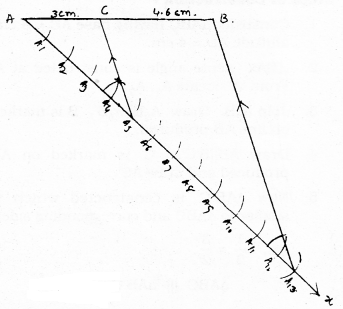Steps of Construction:

1. Draw any ray AB, such that AB = 7.6 cm.
2. Draw Ax ray at point A making acute angle.
3. Locate the points A1, A2, A3, ………., A13 from A.
4. Join BA13. Draw BA13 || A5C.
Now, AC : CB = 5 : 8
If measured, AC = 3 cm, CB = 4.6 cm.

10th Maths Construction Exercise 6.1 Question 2.
Construct a triangle of sides 4 cm, 5 cm and 6 cm and then a triangle similar to it whose sides are 23 of the corresponding sides of the first triangle.
Solution:
Construct a triangle ABC having sides 4 cm, 5 cm and 6 cm. For this we have to construct a triangle similar to it whose sides are 23 of the corresponding sides of the first triangle.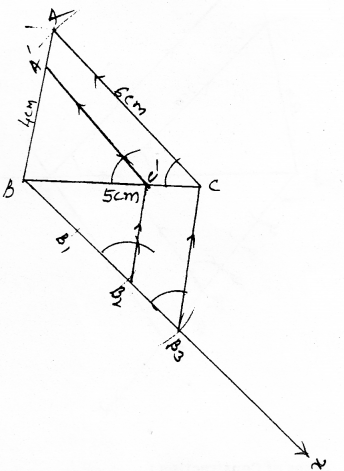Steps of Construction:

1. ∆ABC is constructed, having AB = 4 cm, BC = 5 cm, CA = 6 cm.
2. Draw Bx ray such that it forms acute angle to BC which is adjacent to vertex A.
3. Locate 3 points on Bx such that BB1 = B1B2 = B2B3.
4. Join B3C. Draw a parallel line to B3C which intersect BC at C.
5. Draw a parallel line through C’ to CA and it intersects BA at A’.
Now, A’BC’ is required triangle.

Exercise 6.1 Class 10 Construction Question 3.
Construct a triangle with sides 5 cm, 6 cm and 7 cm and then another triangle whose sides are 75 of the corresponding sides
of the first triangle.
Solution:
Construct an ∆ABC having sides 5 cm, 6 cm and 7 cm. Then construct another triangle whose sides are 75 of the corresponding sides of the first triangle.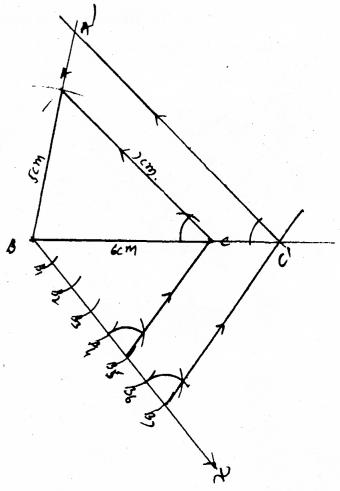Steps of Construction :

1. First construct a triangle ABC having sides AB = 5cm, BC = 6 cm, CA = 7 cm.
2. Draw a ray Bx such that it makes an acute angle at ‘B’.
3. Locate Points B1, B2, B3, B4, B5, B6, By points on Bx.
4. Join B5C. Draw B5C || B7C’, it intersects at ‘C’ which is produced BC line.
5. Draw AC || C’A’, it meets produced line BC at A’.
6. Now required ∆A’BC’ is obtained whose sides are 75 of the corresponding sides of the first triangle.

KSEEB Solutions For Class 10 Maths Construction Question 4.
Construct an isosceles triangle whose base is 8 cm and altitude 4 cm and then another triangle whose sides are 112 times the corresponding sides of the isosceles triangle.
Solution:
Construct an isosceles triangle with base 8 cm and altitude 4 cm and then another triangle whose sides are 112 times the corresponding sides of the isosceles triangle.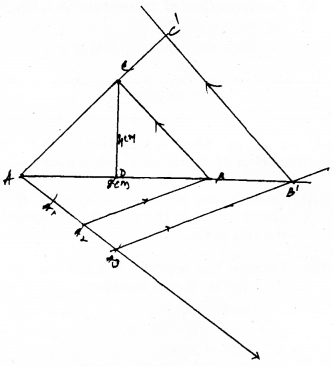Steps of Construction:

1. Construct ∆ABC having base AB = 8 cm, altitude AD = 4 cm.
2. ∠BAx acute angle is constructed at A. From ‘A’ locate A1, A2, A3.
3. Join A2B. Draw A2B || A3B’. B is marked on line AB produced.
4. Draw AB || B’C’. C’ is marked on AC produced and draw AC’.
5. Now ∆AB’C’ is constructed which is similar to ∆ABC and corresponding sides,
i.e., 112=32
∆ABC ||| ∆AB’C’

10th Maths Construction Exercise 6.1 Solutions Question 5.
Draw a triangle ABC with side BC = 6 cm, AB = 5 cm, and ∠ABC = 60°. Then construct a triangle whose sides are 34 of the corresponding sides of the triangle ABC.
Solution:
Construct a triangle ABC with sides BC = 6 cm, AB = 5 cm and ∠ABC = 60°. Then construct a triangle whose sides are 34 of the corresponding sides of the triangle ABC.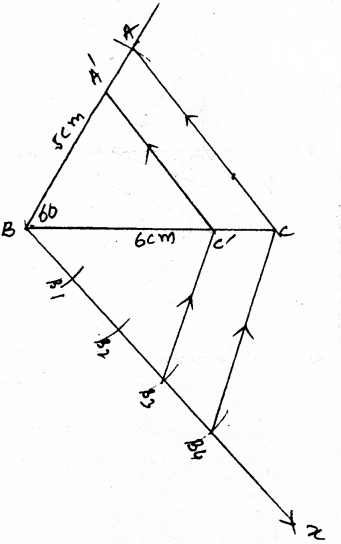Steps of Construction:

1. Construct a AABC having BC = 6 cm, AB = 5 cm, and ∠ABC = 60°.
2. Draw a ray Bx to form acute angle at B and mark BB1, B2, B3, at equal intervals of distance.
3. Join B4C. Draw B4C || B3C’.
4. Draw AC || A’C’.
5. Now ∆ABC’ is equal to ∆ABC.
∴ ∆A’BC’ ||| ∆ABC
3 : 4

Construction Class 10 KSEEB Question 6.
Draw a triangle ABC with side BC = 7 cm, ∠B = 45°, ∠A = 105°. Then, construct a triangle whose sides are 43 times the corresponding sides of ∆ABC
Solution:
Draw a triangle ABC with side BC = cm, ∠B = 45°, ∠A = 105°. The construct a triangle whose sides are 43 times the corresponding sides of ∆ABC.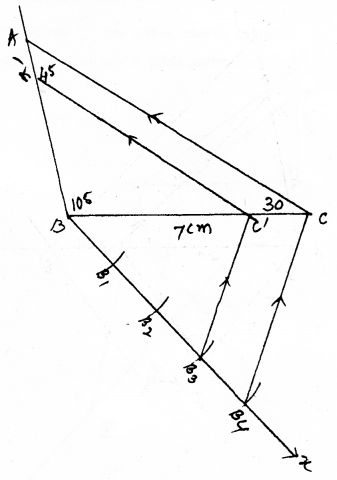Steps of Construction :

1. Construct a triangle ABC having BC = 7 cm, ∠A = 45° and ∠B = 105°.
2. Draw Bx ray at B to form acute angle.
3. Mark points B1, B2, B3, B4 from B. Draw B4C’.
4. Draw B4C || B3C’. Draw AC || A’C’.
5. Now, ∆A’BC’ ||| ∆ABC is constructed.
∆A’BC’ : ∆ABC

Constructions Class 10 Exercise 6.1 Question 7.
Draw a right triangle in which the sides (other than hypotenuse) are of lengths 4 cm and 3 cm. Then construct another triangle whose sides are 53 times the corresponding sides of the given triangle.
Solution:
Draw a right triangle in which the sides (other than hypotenuse) are of lengths 4 cm and 3 cm. Then construct another triangle whose sides are 53 times the corresponding sides of the given triangle.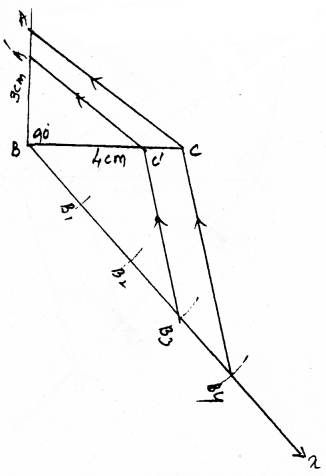Steps of Construction:

1. Construct Right angled triangle ABC having sides BC = 4 cm, BA = 3 cm, and ∠B = 90°.
2. Draw a ray Bx to form acute angle at B.
3. Mark points B1, B2, B3, B4 from B at equal intervals.
4. Join B4C. Draw B4C || B3C’.
5. Draw AC ‘|| C’A’, then ∆A’BC’ is constructed.
6. Now, ∆A’BC’ ||| ∆ABC.
∴ ∆A’BC’ : ∆ABC = 5 : 3.

All Chapter KSEEB Solutions For Class 10 Maths

—————————————————————————–

All Subject KSEEB Solutions For Class 10

*************************************************

I think you got complete solutions for this chapter. If You have any queries regarding this chapter, please comment on the below section our subject teacher will answer you. We tried our best to give complete solutions so you got good marks in your exam.

If these solutions have helped you, you can also share kseebsolutionsfor.com to your friends.

Best of Luck!!